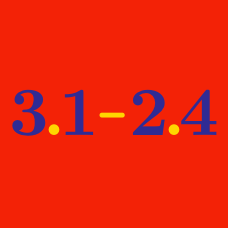Everyday Math

# Decimals - Word Problems

Jenny currently has $15.20$ dollars. She buys $3$ chocolate bars that cost $25$ cents each, and $5$ notebooks that cost 50 cents each. How much does she have left?

A candle has length $24$ cm. Once lit, the candle burns away at a constant rate of $0.2$ cm per minute. What is the length of the candle (in cm) $10$ minutes after it is lit?

James bought $5 \text{ kg}$ of candy for $\ 8.00.$ If Susan wants to buy $1.3 \text{ kg}$ of candy and pays an extra $\ 0.01$ for the paper bag, how much should she pay?

The price for sending a package at the post office varies by the weight of the package according to the following table:

\begin{aligned} \text{Weight of Package (kg)} &&\text{Price (dollars)}\\ \text{Under 1 kg} &&\text{1}\\ \text{1 kg or more and under 3 kg} &&\text{1.5}\\ \text{3 kg or more and under 5 kg} &&\text{2}\\ \text{5 kg or more and under 10 kg} &&\text{2.5}\\ \text{10 kg or more} &&\text{3} \end{aligned}

If Leonard sends a package which consists of one $0.5\text{ kg}$ pen, three $0.1\text{ kg}$ postcards, and two $0.8\text{ kg}$ booklets, how much will he have to pay at the post office?

What is the perimeter of a rectangle whose width is $3.3$ and length is $6.3?$

×